Counting the Tiles of an Interval of the Discrete Line A comment on entries A067824 and A107067 of the Encyclopedia of Integer Sequences

Eric Rivals LIRMM (Computer Science Department) CNRS - Université de Montpellier II Francerivals_AT_lirmm.frhttp://www.lirmm.fr/~rivals

This page (http://www.lirmm.fr/~rivals/RESEARCH/TILING/) is a comment on entries A067824 and A107067 of the Encyclopedia of Integer Sequences .

Our goal was to count the number of tiles of an interval of the discrete line. When we exhibited a close formula for these combinatorial objects, we computed the first terms of the sequence and figured out it already was in the Encyclopedia without any interpretation for it. The sequence was pointing to R. Zumkeller, who gave a program to compute it. So our main result was to give a meaning to this sequence: for an integer n, it is the number of tiles of an interval of length n of the discrete line, Z. We gave two proofs of these results. The second proof shows that two sequences of the Encyclopedia coincide: entries A067824 and A107067, which is our second main result. The latter gives the number of polynomials with coefficients in {0, 1 } that divide the polynomial Xn−1.

This page is also available in PDF format here and the related publication is there, corresponds to reference , which was published in LNCS volume 4009.

1  Introduction

Here, we define the notion of tile. One considers a space to tile, in our case, the space is the discrete line: Z (i.e., {−∞, …, −2, −1, 0, 1, 2, …, +∞}). Let A be a subset of this space. Then A is a tile, if there is another subset B such that A + B = Z, where A + B denotes { a + b : aA, bB }. B is called the dual or the translate of A. B is the set of positions in the space where to put copies of A to cover the whole space. As the definition is symmetrical, B is also a tile.

In general, the sum of two sets yields a multiset, i.e., a set in which some elements occurs more than once. It is not the case for a tile. Thus to denote this instead of A + B = Z one writes:
AB = Z .

1.1  Periodicity of tilings of Z.

Let A, B subsets of Z with A finite. Newman  proved in 1977 that if AB = Z then B is periodic, i.e., it exists kN such that B = k + B . Moreover, he showed that the period k is smaller than 2d( A ), which enables one to check in exponential time whether a pattern A tiles Z, where d( A ) denotes the maximal element of A. However, in all known examples, k ≤ 2d( A ) (Nivat's conjecture) . A periodic tile is given in Example 1.1.

Example 1   The set {0, 1, 4, 5 } tiles Z and its dual is B := { 0, 2} + 8Z.

2  Our case: tiling of an interval of Z.

Let nN with n > 1. We consider the space [[ n ]], i.e., the interval [ 0, n−1 ]. We want to count the number of all possible tiles of [[ n ]] for any n. Let look at some examples.

Example 1
1. Let f = {0,2,4} f =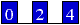f tiles [[ 6 ]] and its dual is f
^6 = {0,1}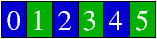Clearly f also tiles Z.

2. Let f = {0, 3, 4, 5, 7, 8} f =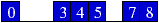f tiles Z with the dual f
^Z= {…, −12, −6, 0, 6, 12, …} = 6Z.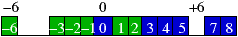f also tiles Z/12Z, but it is not a tile for any interval [[ n ]], whatever n is.

It is thus interesting to distinguish between tiles of Z that also tiles an interval of Z and those that do not.

2.1  Self-periodicity of a tile

We define a notion of self-periodicity of a pattern (a set that is not necessarily a tile). This concept is the pendant of the notion of period for words (or see a text in PDF format). Let n ≥ 0 and f be a pattern such that d( f )<n.

Definition 1   Let p be an integer such that 0 ≤ pd( f ).
p is a
self-period of f for length n if and only if for any i ∈ [0,np[ one has
if ⇔ (i + p) ∈ f .

Let Πn( f ) denotes the set of self-periods of f, and πn( f ) its smallest non null self-period.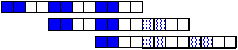2.2  Combinatorial results

We prove that if f is a tile of [[ n ]], and if we denote is dual by f^n then:
• f admits a smallest non null period πn( f )

• πn( f ) divides n and thus, πn( f ) ≤ n/2

• if for an integer m f[m] denotes f[[ m ]], then

fn( f )]   ⊕
 ^ f
n
= [[ πn( f ) ]]

• Theorem 1   There exists a bijection that to any pattern f such that d( f ) ≤ n/2, associates a pattern that tiles [[ d ]] for d a divisor of n.

Example 2   Let n := 12 and f := { 0, 1, 4, 5, 8, 9 }. f tiles [[ 12 ]]; its dual for n := 12 is f^12 := { 0, 2 }.
• f^12 tiles [[ 4 ]], [[ 8 ]], and [[ 12 ]].

• f has periods 0, 4, and 8. So, π12( f ) = 4 and Π12( f ) = { 0, 4, 8 }.

• f can be decomposed in { 0, 1, 4, 5, 8, 9 } = { 0, 1 } ⊕ { 0, 4, 8 }.

• { 0, 1 } and { 0, 4, 8 } are completely periodic for lengths 2 and 12 respectively, with smallest period 1 and 4 respectively.

It means that any tile of the interval [[ n ]] can be decomposed as a sum of completely periodic tile of a shorter interval. This decomposition is also known as Krasner factorization , also see [2, 7] for more information.

2.3  Counting formula

From this, it is possible to enumerate the set, Ξn, of all tiles of [[ n ]] and we obtained a reccurrence formula for ξn, which denotes the number of tiles of [[ n ]], i.e., the cardinality of Ξn.

Theorem 2   One has ξ1 = 1 and
ξn    =    1 +
 Σ d ∈ N : d ∣ n, d ≠ n
ξd  .

Note that if n>1 is prime then Ξn = { { 0 }, [[ n ]] } and
δn = ψn = 1 and ξn = 2.

The values of ξn for n > 0 are those of Sequence entry A067824 in , and (2) corresponds to the recurrence relation given for this sequence by Zumkeller. Using the Moebius inversion, one can easily derive another induction formula for for ξn:

ξn = 1 −
 Σ d|n;d ≠ n
μ (
n
d
)(2ξd − 1)
.

3  Recognition algorithm

We also exhibit an algorithm to recognize if a set f given as input tiles any interval. The algorithm also gives the smallest interval tiled by f, as well as the decomposition of f as a sum of completely self-periodic tiles. It runs in linear time.

Theorem 3   Let f be a pattern. Our algorithm decides in O(d( f )) time whether there is nN such that f tiles [[ n ]] and gives the decomposition of f in completely self-periodic tiles.

Acknowledgents: R. Zumkellerk, O. Gandouet, F. Philippe.

References


Olivier Bodini and Eric Rivals. Tiling an Interval of the Discrete Line. In M. Lewenstein and G. Valiente, editors, Proc. of the 17th Annual Symposium on Combinatorial Pattern Matching (CPM), volume 4009 of Lecture Notes in Computer Science, pages 117–128. Springer Verlag, 2006.


N.G. de Bruijn. On bases for the set of intergers. Publ. Math. Debrecen, 1:232–242, 1950.


M. Krasner and B. Ranulak. Sur une propriété des polynômes de la division du cercle. Comptes rendus de l'Académie des Sciences Paris, 240:397–399, 1937.


J.C. Lagarias and Y. Wang. Tiling the line with translates of one tile. Inventiones Mathematicae, 124(1-3):341–365, 1996.


D.J. Newman. Tesselations of integers. J. Number Theory, 9:107–111, 1977.


N. J. A. Sloane. The On-Line Encyclopedia of Integer Sequences, 2004. Available at https://oeis.org.


R. Tijdeman. Decomposition of the integers as a direct sum of two subsets. In S. David, editor, Number Theory, pages 261–276. Oxford Univ. Press, 1995.

This document was translated from LATEX by HEVEA.